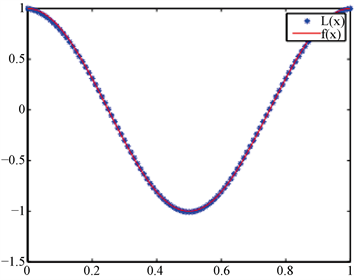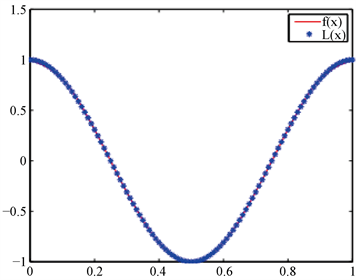# 基于径向基函数插值的非线性积分方程求解Nonlinear Integral Equation Solution Based on Radial Basis Function Interpolation

DOI: 10.12677/AAM.2019.811209, PDF, HTML, XML, 下载: 295  浏览: 541  国家自然科学基金支持

Abstract: The radial basis function (RBF) interpolation is applied to the solution of the nonlinear integral equation. In this paper, the multiquadric (MQ) function with excellent interpolation performance in RBF is selected. First, the function to be solved is expressed as a linear combination of RBF, and then the equation is discretized into a non-linear square by collocation method. The approximate expression of the function to be solved is given after the weight coefficients obtained. Solving nonlinear integral equation based on MQ function interpolation can obtain higher accuracy with fewer nodes, which can be extended to high-dimensional integral equation. In this paper, we prove the existence and uniqueness of MQ basis function interpolation problem, and analyse the interpolation basis function of constructing non-linear function of MQ function by numerical examples, and obtain the ideal approximation effect and result.

1. 引言

$f\left(P\right)=\mu {\int }_{\Omega }k\left(P,Q\right)F\left[f\left(Q\right)\right]\text{d}Q+g\left(P\right),\text{\hspace{0.17em}}\text{\hspace{0.17em}}P,Q\in \Omega$ (1)

2. 基于RBF插值改进的配置法算法

2.1. RBF插值原理

$\Phi \left(x\right)={\int }_{{\Re }^{l}}{\text{e}}^{ixt}\stackrel{^}{\Phi }\left(t\right)\text{d}t$，当 ${x}_{j}$ 两两不同且 ${\lambda }_{j}$ 不全为零时，有

$\underset{j,k=1}{\overset{n}{\sum }}{\lambda }_{j}{\lambda }_{k}\Phi \left(‖x‖\right)={\int }_{{\Re }^{l}}|\underset{j}{\overset{n}{\sum }}{\lambda }_{j}{\text{e}}^{ix{j}^{t}}|\stackrel{^}{\Phi }\left(t\right)\text{d}t$ (2)

(1) $\psi \left(r\right)\in C\left[0,\infty \right]$

(2) $\psi \left(r\right)\in {C}^{\infty }\left[0,\infty \right]$

(3) ${\left(-1\right)}^{k}{\psi }^{k}\left(r\right)>0,\text{\hspace{0.17em}}r>0,\text{\hspace{0.17em}}k=0,1,2,\cdots$

$\underset{j,k=1}{\overset{n}{\sum }}{\lambda }_{j}{\lambda }_{k}\Phi \left(‖{x}_{k}-{x}_{j}‖\right)={\int }_{{\Re }^{l}}|\underset{j=1}{\overset{n}{\sum }}{\lambda }_{j}{\text{e}}^{ix{j}^{t}}|\stackrel{^}{\Phi }\left(t\right)\text{d}t>0$ (3)

$A=\left[\begin{array}{cc}\Phi \left(‖{x}_{j}-{x}_{k}‖\right)& \left({x}_{j}^{\beta }\right)\\ {\left({x}_{j}^{\beta }\right)}^{\text{T}}& 0\end{array}\right]$

${f}^{*}\left(x\right)=\underset{j=1}{\overset{n}{\sum }}{a}_{j}\Phi \left(‖x-{x}_{j}‖\right)+{\underset{|\beta | (4)

$f\left(x\right)={f}^{*}\left({x}_{k}\right),k=1,2,\cdots ,n$ (5)

2.2. 基于RBF插值的改进配置法算法

RBF是仅依赖于距离 $r=‖x-{x}_{j}‖$ 的函数，在多种RBF的定义中，常用的有薄板样条函数 $\Phi \left(r\right)={r}^{2}\mathrm{ln}r$ 高斯函数 $\Phi \left(r\right)={\text{e}}^{\beta {r}^{2}}$ 、Hardy提岀的多重二次曲面(multi-quadric, MQ)函数及多种紧支撑函数等。Franke在1982年证明了MQ方法是所有插值方法中综合性能最优的，因此本文选用MQ作为插值基函数，其常用表达式为

$\Phi \left(x\right)=\sqrt{{‖x-c‖}^{2}+{a}^{2}}$ (6)

Step 1：设置RBF函数的中心点c，形状参数 $\alpha \left(\alpha \in \left(0,1\right]\right)$，配置点 ${x}_{m},\text{\hspace{0.17em}}m=1,2,\cdots ,N$

Step 2：对给定的一组数据点 $\left({x}_{m},f\left({x}_{m}\right)\right)$，由RBF构造插值函数

$f\left(x\right)=\underset{i=1}{\overset{N}{\sum }}{\lambda }_{i}{\Phi }_{i}\left(x\right)=\underset{i=1}{\overset{N}{\sum }}{\lambda }_{i}\sqrt{{\left(x-{c}_{i}\right)}^{2}+{\alpha }^{\text{2}}}$ (7)

Step 3：将插值点代入(7)式，得

$f\left({x}_{m}\right)=\underset{i=1}{\overset{N}{\sum }}{\lambda }_{i}{\Phi }_{i}\left({x}_{m}\right)=\underset{i=1}{\overset{N}{\sum }}{\lambda }_{i}\sqrt{{\left({x}_{m}-{c}_{i}\right)}^{2}+{\alpha }^{2}}$ (8)

$\left[{\Phi }_{d}\right]\left[\lambda \right]=\left[{f}_{d}\right]$ (9)

Step 4: 矩阵元素计算式为 ${\Phi }_{mn}=\sqrt{{\left({x}_{m}-{c}_{n}\right)}^{2}+{\alpha }^{2}}$，求解(9)式得

$\left[\lambda \right]={\left[{\Phi }_{d}\right]}^{-1}\left[{f}_{d}\right]$ (10)

Step 5: 得岀函数 $f\left(x\right)$ 的RBF逼近形式

$f\left(x\right)=\left[\Phi \left(x\right)\right]\left[\lambda \right]=\left[\Phi \left(x\right)\right]{\left[{\Phi }_{d}\right]}^{-1}\left[{f}_{d}\right]$ (11)

$S\left(x\right)={\sum }_{j=1}^{n}\Phi \left(‖{x}_{k}-{x}_{j}‖\right)$

$S\left({x}_{k}\right)=\underset{j=1}{\overset{n}{\sum }}{\lambda }_{j}\Phi \left(‖{x}_{k}-{x}_{j}‖\right)={f}_{k},k=1,2,\cdots ,n$

$\sum \sum {\lambda }_{j}{\lambda }_{k}{\text{e}}^{-{‖{x}_{j}-{x}_{k}‖}^{2}}={\left(2\pi \right)}^{d/2}\int {|{\lambda }_{j}{\text{e}}^{ix{j}^{\theta }}|}^{2}{\text{e}}^{-{‖\theta ‖}^{1/2}}\text{d}\theta >0$

$\begin{array}{l}\sum {\lambda }_{j}{\lambda }_{k}{\int }_{0}^{\infty }{\theta }^{-3/2}\left(1-{\text{e}}^{-\theta \left({\alpha }^{2}+{‖x‖}^{2}\right)}\right)\text{d}\theta \\ =\sum {\lambda }_{j}{\lambda }_{k}{\left({\alpha }^{2}+{‖{x}_{k}-{x}_{j}‖}^{2}\right)}^{1/2}{\int }_{0}^{\infty }{\theta }^{-3/2}\left(1-{\text{e}}^{-\theta }\right)\text{d}\theta \\ =h\sum {\lambda }_{j}{\lambda }_{k}{\left({\alpha }^{2}+{‖{x}_{k}-{x}_{j}‖}^{2}\right)}^{1/2}\\ <0\end{array}$

$\sum {\lambda }_{j}{\lambda }_{k}{\left({\alpha }^{2}+{‖{x}_{k}-{x}_{j}‖}^{2}\right)}^{1/2}<0$

$\sum {\lambda }_{j}{\lambda }_{k}{\left({\alpha }^{2}+{‖{x}_{k}-{x}_{j}‖}^{2}\right)}^{1/2}={\sum \left({\alpha }^{2}+{‖{x}_{k}-{x}_{j}‖}^{2}\right)}^{1/2}>0$

4. 数值实验及分析

$L\left(x\right)=\underset{j=0}{\overset{N}{\sum }}{a}_{j}{\Phi }_{j}\left(x\right)$(12)

$L\left({x}_{k}\right)=\underset{j=0}{\overset{N}{\sum }}{a}_{j}{\Phi }_{j}\left({x}_{k}\right)=\underset{j=0}{\overset{N}{\sum }}{a}_{j}\left({x}_{j}-{x}_{k}\right)=f\left({x}_{k}\right)$ (13)

$\Phi \left({x}_{j}-{x}_{k}\right)=\sqrt{{\alpha }^{2}+{\left({x}_{j}-{x}_{k}\right)}^{2}}\left(k=0,1,\cdots ,N;j=0,1,\cdots ,N\right)$ 非奇异，故此方程组有唯一解，可求出 ${a}_{j}$，代入(12)式得到 $L\left(x\right)$，进而求出插值函数。下面给出了误差表1及两种插值图1图2Figure 1. $N=6$, $L\left(x\right),f\left(x\right)$ function imageFigure 2. $N=11$, $L\left(x\right),f\left(x\right)$ function imageTable 1. The maximum error comparison between the method in this paper and the methods in   

5. 结论

NOTES

*通讯作者。